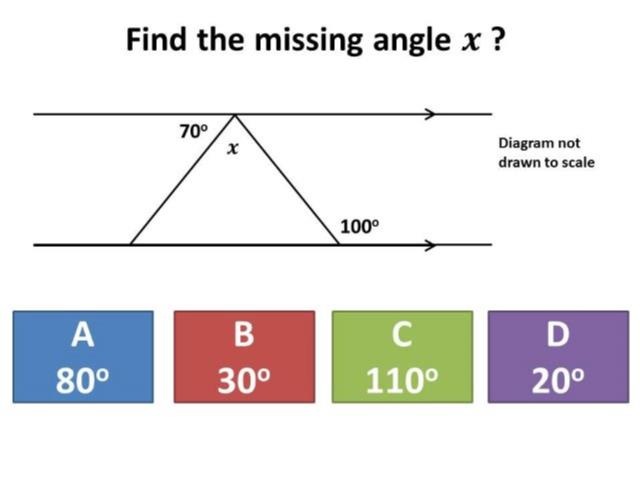# Angle Facts – The Answers Revealed!

It has been a dramatic week for Guess the Misconception, upon its return after the Easter break.

Firstly, there has been an outcry, both in my maths department and across the nation as a whole, from teachers asking “why wasn’t 100 one of the answers?” or “what happened to 40?”. This highlights both an inherent problem with multiple choice questions – namely, what happens if the answer a student thinks is correct is not actually one of the given answers? – but also reinforces the importance of students giving explanations to support their answer on Diagnostic Questions. A few of our Year 11s gave explanations such as “I am going for 110, but I reckon the answer is 100 due to alternate angles being equal”. This allowed us to go beyond the data and understand this student’s misconceptions.

Secondly – and possibly even more dramatically – the least popular misconception as voted for by teachers, turned out to be the most popular misconception held by students!

This question in… well, question… on angle facts is one of the most poorly answered across the free daily GCSE Revision Streamto date, with over half of students getting the answer wrong. Let’s take a look at the question, and see the kind of misconceptions that it reveals:Teachers felt that A would be the most common choice, and indeed this did attract around 30% of incorrect student answers, with explanations revealing misconceptions around straight lines and the type of triangle invovled, such as:

“It’s a as it sits on a straight line and angles on a straight line add up to 180 degrees so if you take it away from 180 take away from 100 it’s left with 80″

“A straight line is 180° And this triangle is an equilateral triangle meaning all angles are the same size, triangle is 180°. 180-100 = 80, meaning the other two angles are also 80°”

But the answer that attracted over 40% of incorrect student responses was D. Can you see why? Let’s read some of explanations from the thousands of students who opted for this to find out:

“Exterior angle on the bottom two corners are 100 degrees, the angle of a straight line is 180 degrees so 180-100=80 times that by two equals 160 and then take 160 from 180 as that’s the angles in a triangle added together and that equals 20″

“This is an isosceles triangle meaning that the 2 bottom angles are equal. So first you have to do 180-100 because angles in a straight line and you get 80. This means the 2 bottom angles are going to be 80 degrees. After to find the top angle I did 80+80 which is 160 and then take that away from 180 and you get 20 degrees.”

“because the angle labelled 100 degrees is on a straight time which sums to 180 and that means the angle beside it is 80 degrees and both bottom interior angles are the same so 80 plus 80 is 160 and all interior angles in a triangle make 180 so you do 180 minus 160 which is 20 degrees.”

“First work out The angles along the bottom line 180 degrees on a straight line, so 180 – 100 (which is the exterior degree) = 80 so the angle to the bottom right is 80 degrees, as is the one on the left Inside a triangle the angles add to 180 There are already two 80 degrees 80 + 80 = 160 180-160 = 20 degrees”

What do we take from this? Well, as a maths department we were a little shocked firstly at how poorly our Year 11s performed on this question, but also the range of the misconceptions they held. Some teachers decided they were going to show this question at the start of the next lesson and allow students to discuss the answers. Other teachers announced they were doing a full angle facts revision lesson. But all decided that some sort of action was required. This might be the case with your Year 11s as wellWith the GCSE Maths exams getting closer, now is the perfect time to sign your Year 11s students up to the free daily GCSE Revision Stream. Over 10,000 students around the country cannot be wrong